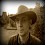## Wednesday, September 19, 2012

### No half a bit of info

I argue below that A photon is not a math object. I elaborate with a simple analogy.

Say I have a piece of paper with a 0 and 1 on it. I tear it in half, and mail the pieces to London and Australia, so one gets a 0 and one gets a 1. How many bits of info are in the London envelope? One, of course, as it has a 0 or a 1. How many in the Australiz envelope? Also one, but now it gets tricky because we don't have two bits of info overall.

So there is just one bit of info overall, but it does not make much mathematical sense to say that each envelope has half a bit of info. What is half a bit? It seems like a whole bit to the guy in London.

The spin of an entangled electron pair is similar. You can separate the electrons and find that each has a spin that is easily understandable by itself. But somehow the pair has a collective spin that is not so easily divided into two spins.

When the guy in London opens the envelope, he does not have any spooky nonlocal effect on the Australia envelope. There is nothing mysterious at all. You could explain it to a child. It only gets tricky when you try to apply certain mathematical quantifications, such as asking how many bits of info are in the London envelope, or asking what the probability is of a 1 in that envelope. (It seems like a probability of 0.5, but the answer seems to change as the Australian envelope gets opened.)

Likewise, the physics of entangled electrons is not tricky or mysterious. It only gets tricky when you try to match up the physics with mathematical quantifications of spin.

I say that the best explanation is that the electron does not have an adequate mathematical quantification. Just like the London envelope.

This would all be fairly trivial except for physicists who insist on saying all sorts of crazy things about the entangled electron. They say that the electron has a spooky effect on distant electrons, or that there is no reality. And they say those crazy things because they assume that the electron has a state that is completely characterized by mathematical quantifications.

1.I have no idea what you're trying to say here. It's very easy to represent a pair of entangled electrons mathematically. You just need to use the appropriate mathematical concept for measuring information in a quantum mechanical system:

http://en.wikipedia.org/wiki/Qubit

2.Yes, that article gives the Bell state as a mathematical representation of the entangled pair of electrons. But there is no such representation of an individual electron.

3.Well, I've told you before what I think of that argument. It's like saying the trajectory of a golf ball has no mathematical explanation if you ignore all the forces acting on the ball...

4.The trajectory of a golf ball is explainable in terms of a mathematical description of the ball and the fields and molecules surrounding the ball. The same cannot be said for an entangled electron.

5.This isn't my field, but for me your analogy is very useful in trying to make sense of the things physicists do in their experiments as against the mystifying things many of them say.